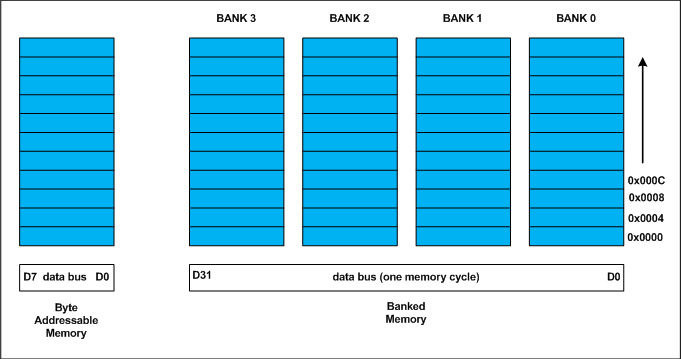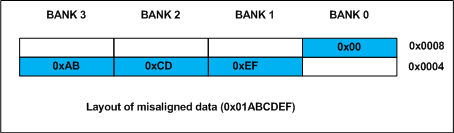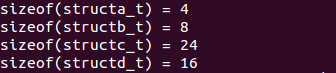字节对齐

分析

Every data type in C/C++ will have alignment requirement (infact it is mandated by processor architecture, not by language). A processor will have processing word length as that of data bus size. On a 32 bit machine, the processing word size will be 4 bytes.Historically memory is byte addressable and arranged sequentially. If the memory is arranged as single bank of one byte width, the processor needs to issue 4 memory read cycles to fetch an integer. It is more economical to read all 4 bytes of integer in one memory cycle. To take such advantage, the memory will be arranged as group of 4 banks as shown in the above figure.

The memory addressing still be sequential. If bank 0 occupies an address X, bank 1, bank 2 and bank 3 will be at (X + 1), (X + 2) and (X + 3) addresses. If an integer of 4 bytes is allocated on X address (X is multiple of 4), the processor needs only one memory cycle to read entire integer.

Where as, if the integer is allocated at an address other than multiple of 4, it spans across two rows of the banks as shown in the below figure. Such an integer requires two memory read cycle to fetch the data.A variable’s data alignment deals with the way the data stored in these banks. For example, the natural alignment of int on 32-bit machine is 4 bytes. When a data type is naturally aligned, the CPU fetches it in minimum read cycles.

Similarly, the natural alignment of short is 2 bytes. It means, a short can be stored in bank 0 – bank 1 pair or bank 2 – bank 3 pair. A double requires 8 bytes, and occupies two rows in the memory banks. Any misalignment of double will force more than two read cycles to fetch double data.

Note that a double variable will be allocated on 8 byte boundary on 32 bit machine and requires two memory read cycles. On a 64 bit machine, based on number of banks, double variable will be allocated on 8 byte boundary and requires only one memory read cycle.

题解

For the sake of convenience, assume every structure type variable is allocated on 4 byte boundary (say 0x0000), i.e. the base address of structure is multiple of 4 (need not necessary always, see explanation of structc_t).

structure A

The structa_t first element is char which is one byte aligned, followed by short. short is 2 byte aligned. If the the short element is immediately allocated after the char element, it will start at an odd address boundary. The compiler will insert a padding byte after the char to ensure short will have an address multiple of 2 (i.e. 2 byte aligned). The total size of structa_t will be sizeof(char) + 1 (padding) + sizeof(short), 1 + 1 + 2 = 4 bytes.

structure B

The first member of structb_t is short followed by char. Since char can be on any byte boundary no padding required in between short and char, on total they occupy 3 bytes. The next member is int. If the int is allocated immediately, it will start at an odd byte boundary. We need 1 byte padding after the char member to make the address of next int member is 4 byte aligned. On total, the structb_t requires 2 + 1 + 1 (padding) + 4 = 8 bytes.

structure C – Every structure will also have alignment requirements

Applying same analysis, structc_t needs sizeof(char) + 7 byte padding + sizeof(double) + sizeof(int) = 1 + 7 + 8 + 4 = 20 bytes. However, the sizeof(structc_t) will be 24 bytes. It is because, along with structure members, structure type variables will also have natural alignment. Let us understand it by an example. Say, we declared an array of structc_t as shown below

Assume, the base address of structc_array is 0x0000 for easy calculations. If the structc_t occupies 20 (0x14) bytes as we calculated, the second structc_t array element (indexed at 1) will be at 0x0000 + 0x0014 = 0x0014. It is the start address of index 1 element of array. The double member of this structc_t will be allocated on 0x0014 + 0x1 + 0x7 = 0x001C (decimal 28) which is not multiple of 8 and conflicting with the alignment requirements of double. As we mentioned on the top, the alignment requirement of double is 8 bytes.

Inorder to avoid such misalignment, compiler will introduce alignment requirement to every structure. It will be as that of the largest member of the structure. In our case alignment of structa_t is 2, structb_t is 4 and structc_t is 8. If we need nested structures, the size of largest inner structure will be the alignment of immediate larger structure.

In structc_t of the above program, there will be padding of 4 bytes after int member to make the structure size multiple of its alignment. Thus the sizeof (structc_t) is 24 bytes. It guarantees correct alignment even in arrays. You can cross check.

structure D – How to Reduce Padding?

By now, it may be clear that padding is unavoidable. There is a way to minimize padding. The programmer should declare the structure members in their increasing/decreasing order of size. An example is structd_t given in our code, whose size is 16 bytes in lieu of 24 bytes of structc_t.

答案结论

１:数据成员对齐规则：结构(struct)(或联合(union))的数据成员，第一个数据成员放在offset为0的地方，以后每个数据成员存储的起始位置要从该成员大小或者成员的子成员大小（只要该成员有子成员，比如说是数组，结构体等）的整数倍开始(比如int在32位机为４字节,则要从４的整数倍地址开始存储)。

２:结构体作为成员:如果一个结构里有某些结构体成员,则结构体成员要从其内部最大元素大小的整数倍地址开始存储。

３:收尾工作:结构体的总大小,也就是sizeof的结果,必须是其内部最大成员的整数倍,不足的要补齐。